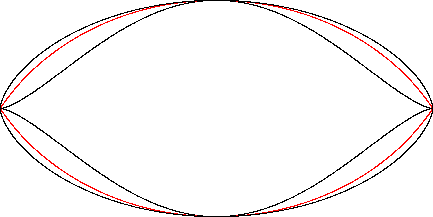Vesica Piscis
VESICA PISCIS

Balmoral Software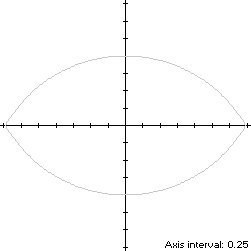The Vesica Piscis is the convex intersection of two congruent circles that pass through each other's center. Here we take the common radius as 2 and the centers on the y-axis at (0,±1). Since each circle from which S is constructed is symmetric with respect to the y-axis and each is reflected across the x-axis from the other, S is bisymmetric. In order for S to be traversed in a counterclockwise direction around the origin starting from its right-hand corner, its parametric equations are:The abscissa extrema of S are at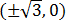and the ordinate extrema at the two circle centers (0,±1), so the width x height of the bounding rectangle of S is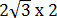.

### Metrics

The perimeter of the Vesica Piscis is 8π/3 and its area is### Boundary Circles & Circumellipse

The squared distance function x2(t) + y2(t) of S ranges from a minimum of 1 at (0,±1) to a maximum of 3 at, so the inradius is 1 and the circumradius is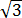. Since these extrema occur on the coordinate axes, a candidate for the circumellipse is one enclosed by the annulus between the two boundary circles, with a =and b = 1. For verification, we have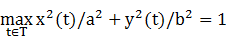### Inellipse

In Lemma B, we have for the upper arch
x(t)y(t) = 2cos(2t/3 + π/6)[2sin(2t/3 + π/6) - 1]
This expression is maximized in the first quadrant at the complicated value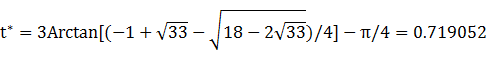The circumellipse dimensions are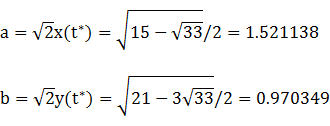For verification, we have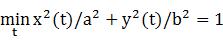### Summary Table

 Perimeter Area Centroid Figure Parameters Incircle R = 1 6.283185 3.141593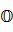Inellipse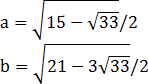7.923165 4.637100 Vesica Piscis8.377580 4.913479 Circumellipse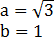8.737753 5.441399 Circumcircle R =10.882796 9.424778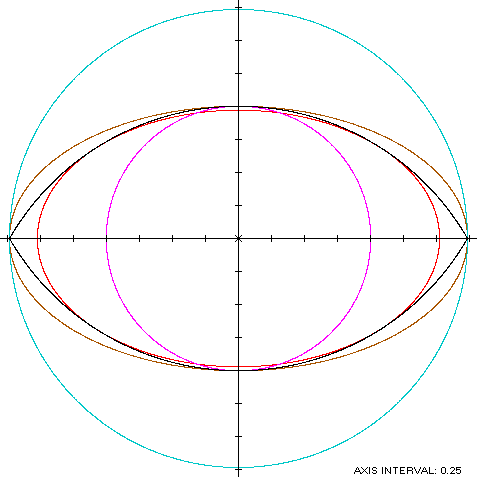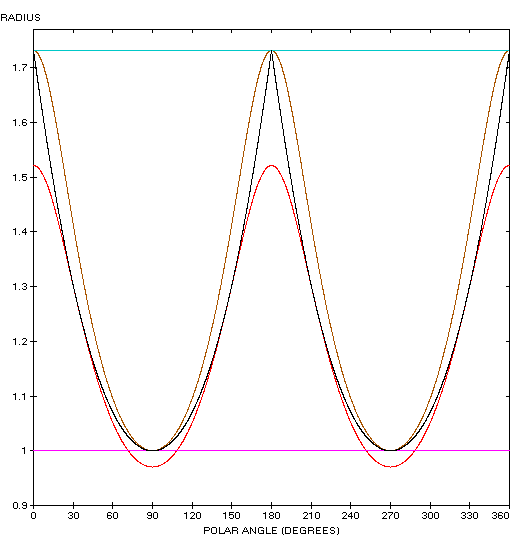"The CBS Television Network"

The Vesica Piscis (red) is a member of a group of similarly-shaped figures described on these pages, including (inside to outside) the mouth curve and the cycloid: